# P Block - Group 15 Questions and Answers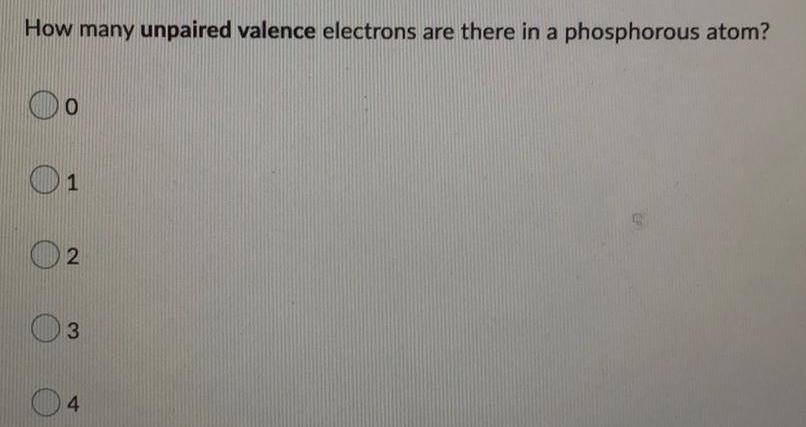Inorganic Chemistry
P Block - Group 15
How many unpaired valence electrons are there in a phosphorous atom? 0 1 2 3 4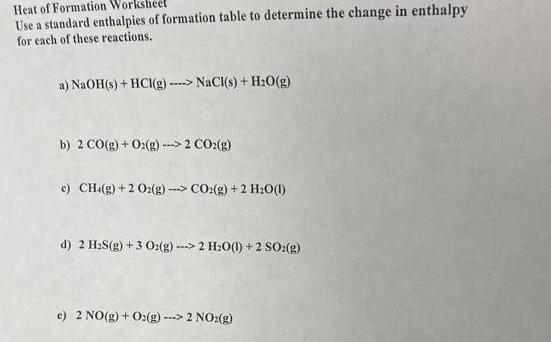Inorganic Chemistry
P Block - Group 15
Heat of Formation Worksheet Use a standard enthalpies of formation table to determine the change in enthalpy for each of these reactions. a) NaOH(s) + HCl(g) ----> NaCl(s) + H₂O(g) b) 2 CO(g) + O2(g) ---> 2 CO₂(g) c) CH4(g) + 2 O2(g)---> CO₂(g) + 2 H₂O(l) d) 2 H₂S(g) + 3 O2(g) ---> 2 H₂O(1) +2 SO2(g) e) 2 NO(g) + O2(g) ---> 2 NO₂(g)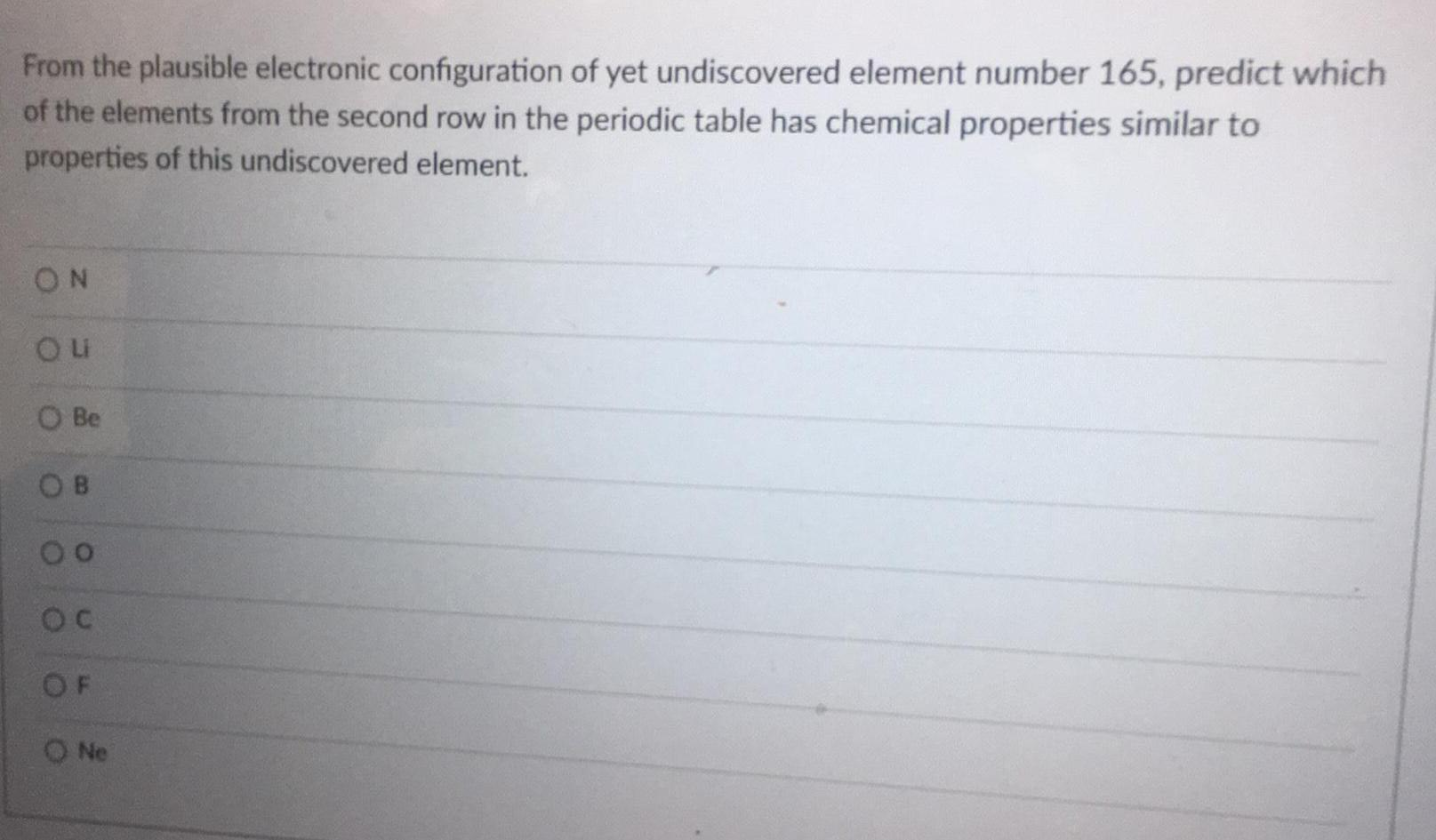Inorganic Chemistry
P Block - Group 15
From the plausible electronic configuration of yet undiscovered element number 165, predict which of the elements from the second row in the periodic table has chemical properties similar to properties of this undiscovered element. N U Be C O F Ne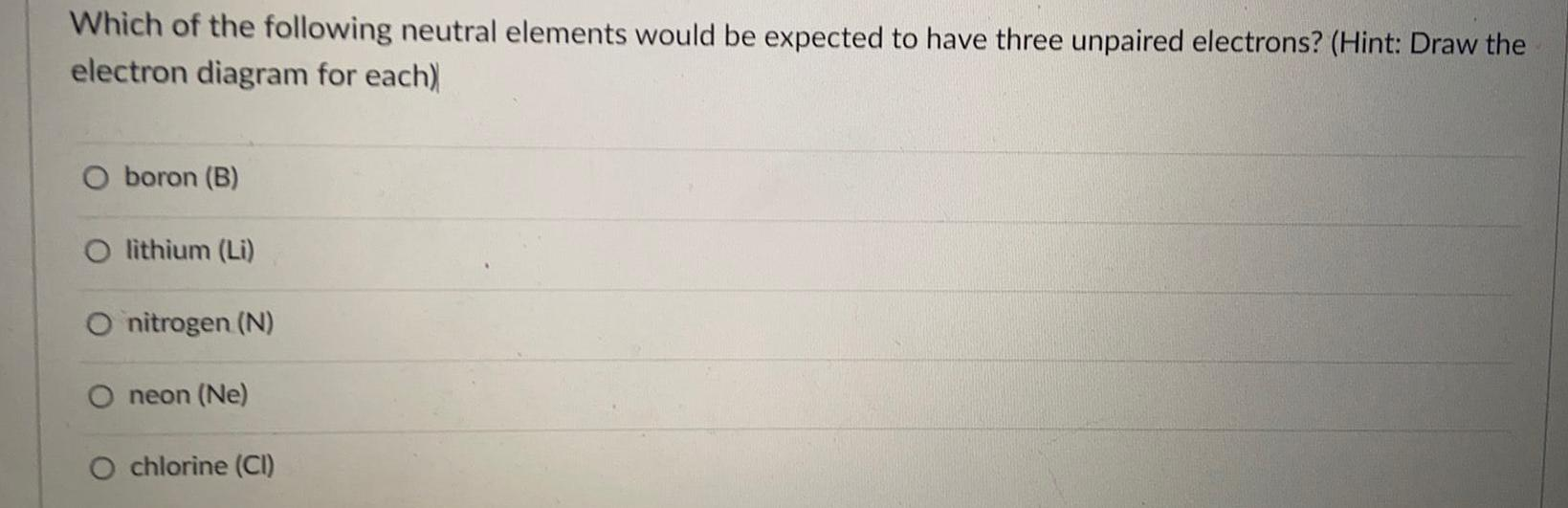Inorganic Chemistry
P Block - Group 15
Which of the following neutral elements would be expected to have three unpaired electrons? (Hint: Draw the electron diagram for each) boron (B) lithium (Li) nitrogen (N) neon (Ne) chlorine (CI)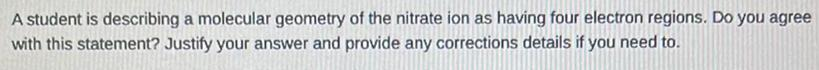Inorganic Chemistry
P Block - Group 15
A student is describing a molecular geometry of the nitrate ion as having four electron regions. Do you agree with this statement? Justify your answer and provide any corrections details if you need to.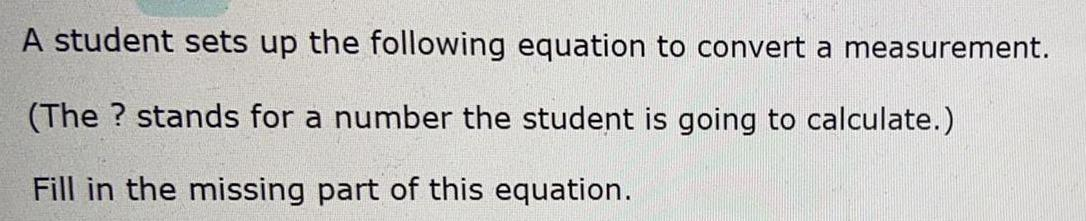Inorganic Chemistry
P Block - Group 15
A student sets up the following equation to convert a measurement. (The? stands for a number the student is going to calculate.) Fill in the missing part of this equation.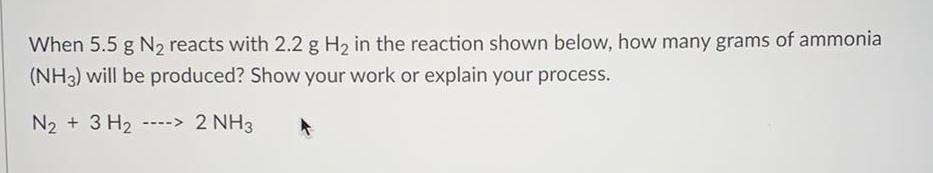Inorganic Chemistry
P Block - Group 15
When 5.5 g N₂ reacts with 2.2 g H₂ in the reaction shown below, how many grams of ammonia (NH3) will be produced? Show your work or explain your process. N₂ + 3 H₂ --> 2 NH3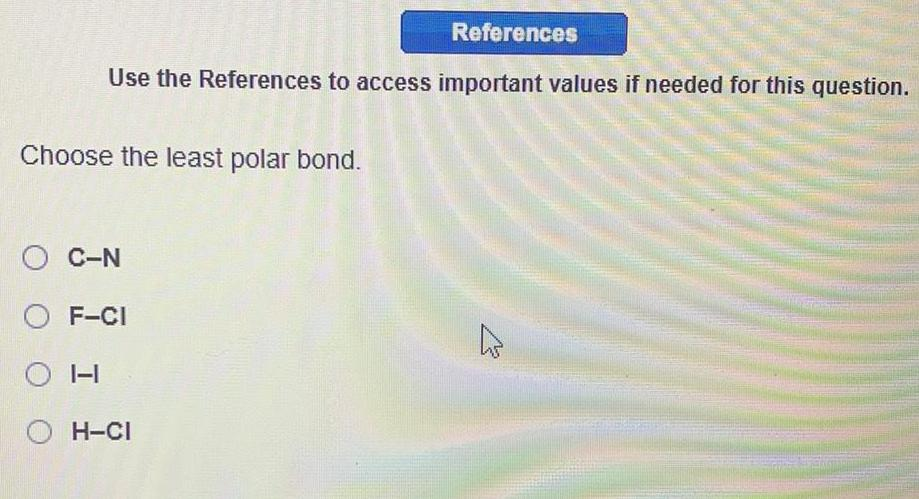Inorganic Chemistry
P Block - Group 15
Choose the least polar bond. C-N F-CI OH H-CI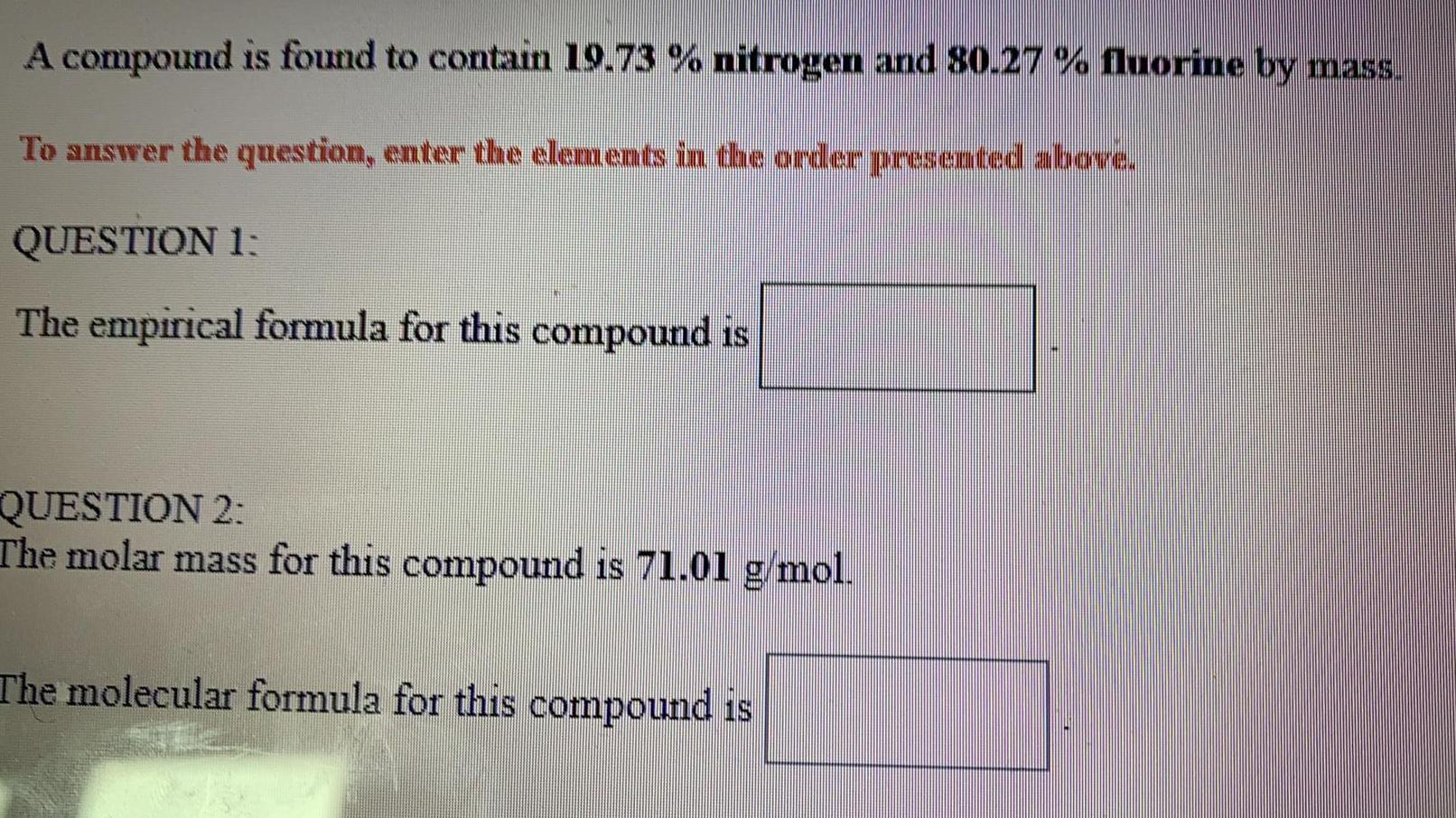Inorganic Chemistry
P Block - Group 15
A compound is found to contain 19.73 % nitrogen and 80.27 % fluorine by mass. To answer the question, enter the elements in the order presented above. QUESTION 1: The empirical formula for this compound is QUESTION 2: The molar mass for this compound is 71.01 g/mol. The molecular formula for this compound is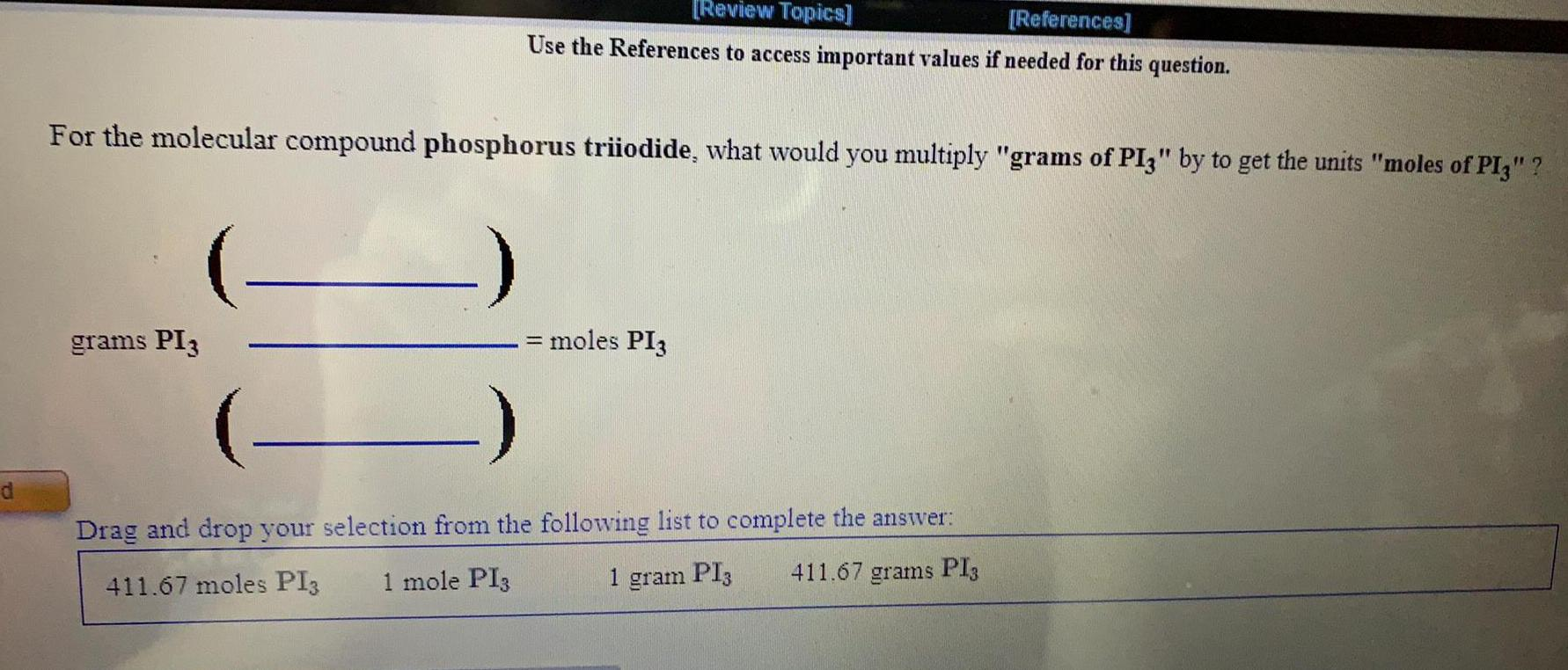Inorganic Chemistry
P Block - Group 15
For the molecular compound phosphorus triiodide, what would you multiply "grams of PI3" by to get the units "moles of PI3" ? grams PI3 ?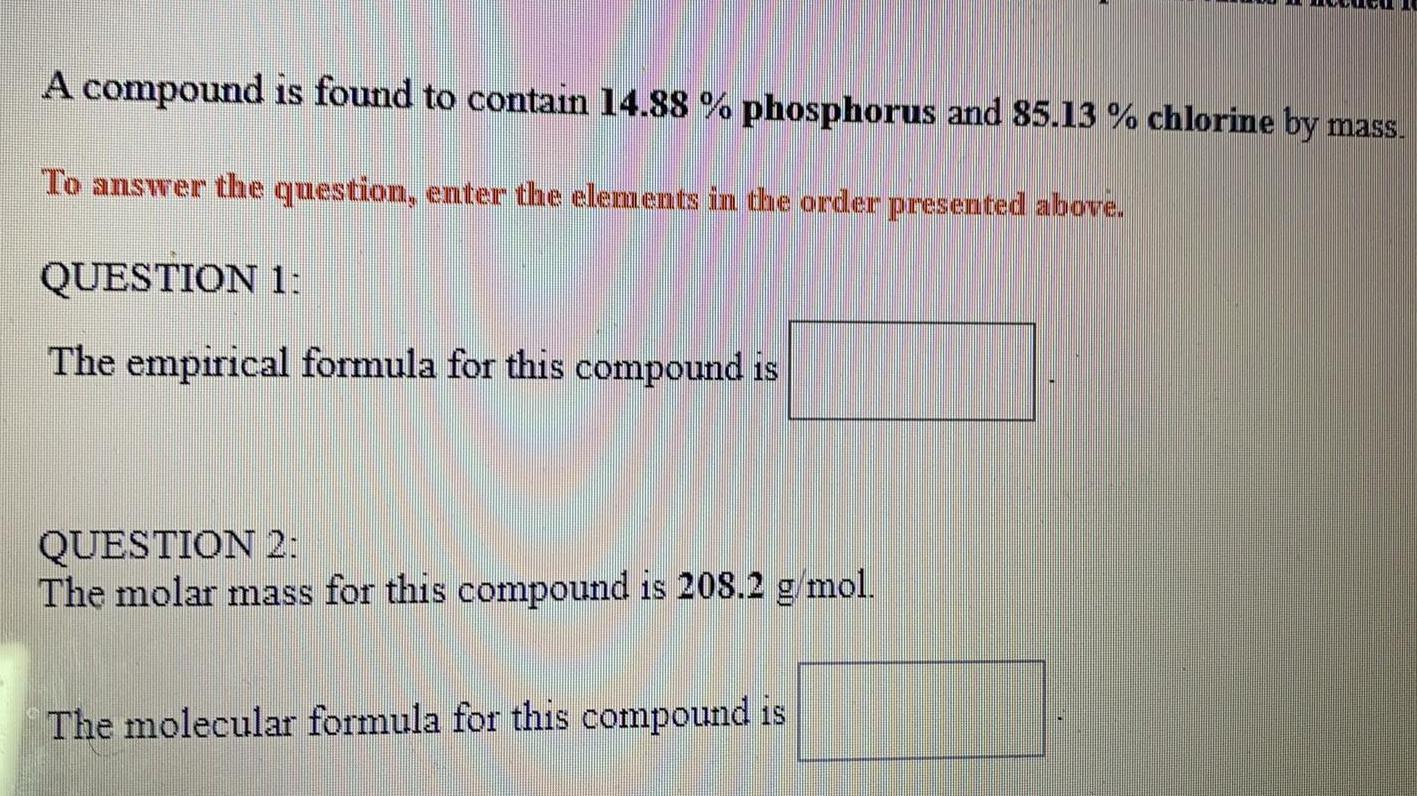Inorganic Chemistry
P Block - Group 15
A compound is found to contain 14.88 % phosphorus and 85.13 % chlorine by mass. . QUESTION 1: The empirical formula for this compound is_____? QUESTION 2: The molar mass for this compound is 208.2 g/mol. The molecular formula for this compound is_____?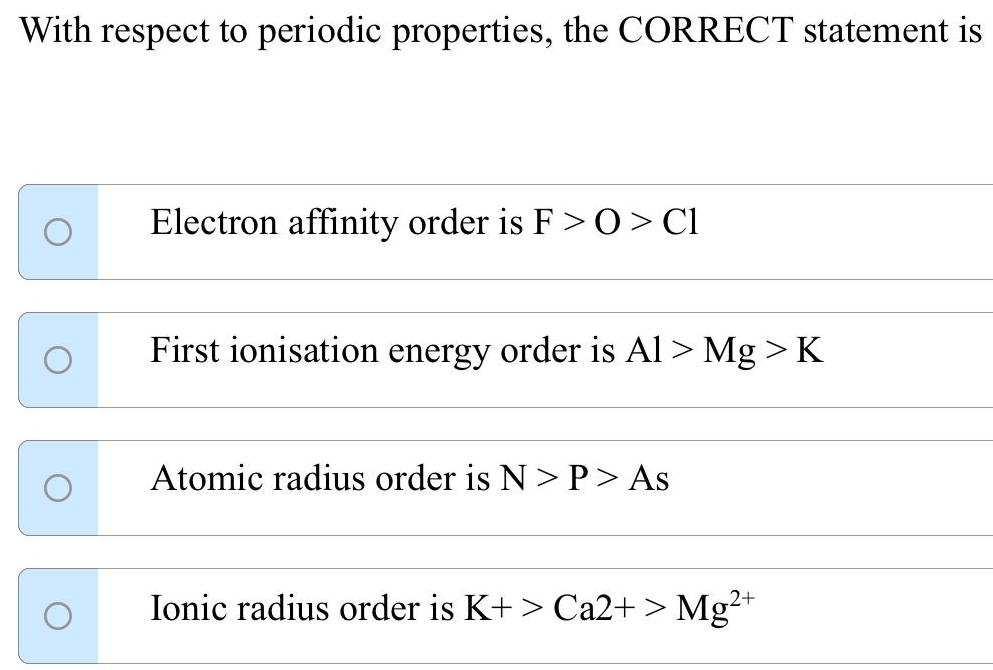Inorganic Chemistry
P Block - Group 15
With respect to periodic properties, the CORRECT statement is Electron affinity order is F>O > Cl First ionisation energy order is Al> Mg > K Atomic radius order is N> P > As Ionic radius order is K+ > Ca2+ > Mg²+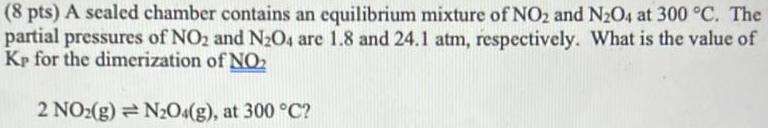Inorganic Chemistry
P Block - Group 15
A sealed chamber contains an equilibrium mixture of NO₂ and N₂O4 at 300 °C. The partial pressures of NO2 and N2O4 are 1.8 and 24.1 atm, respectively. What is the value of Kp for the dimerization of NO2 2 NO₂(g) = N2O4(g), at 300 °C?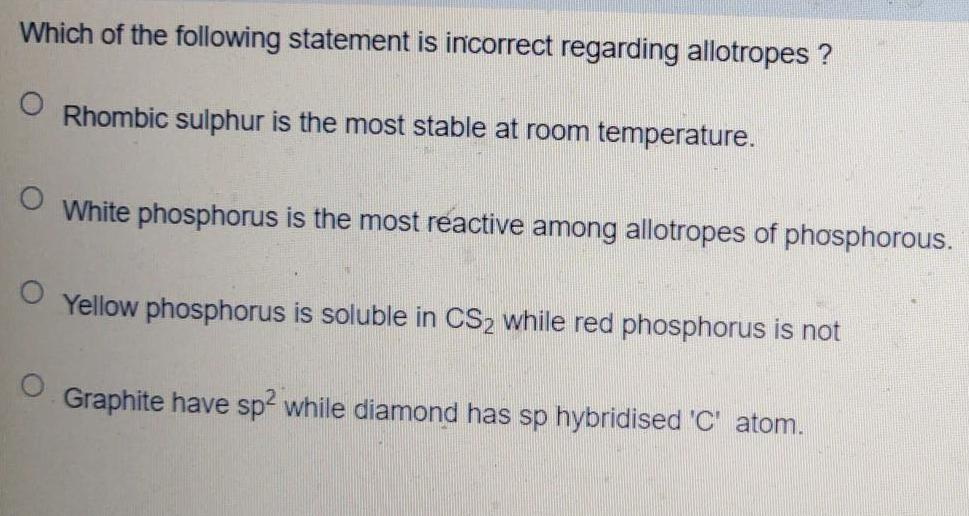Inorganic Chemistry
P Block - Group 15
Which of the following statement is incorrect regarding allotropes ? Rhombic sulphur is the most stable at room temperature. White phosphorus is the most reactive among allotropes of phosphorous. Yellow phosphorus is soluble in CS₂ while red phosphorus is not Graphite have sp2 while diamond has sp hybridised 'C' atom.
1